## Method

efficient Visual Odometer [st] [eVO]

Submitted on 6 Mar. 2013 18:49 by
Martial Sanfourche (ONERA)

 Running time: 0.05 s Environment: 2 cores @ 2.0 Ghz (C/C++)

 Method Description: Pragramatic stereo visual odometer based on sparse features tracking. Parameters: 480 Harris points par view spatially spreadKeyframe regeneration parameter = 75% Latex Bibtex: @InProceedings{Sanfourche13,author = {M. Sanfourche and V. Vittori and G. Le Besnerais},title = {eVO: A realtime embedded stereo odometry for MAV applications},booktitle = {IEEE/RSJ International Conference on Intelligent Robots and Systems (IROS)},OPTcrossref = {•},OPTkey = {•},pages = {2107-2114},year = {2013},OPTeditor = {•},OPTvolume = {•},OPTnumber = {•},OPTseries = {•},address = {Tokyo, Japan},month = {November},OPTorganization = {•},OPTpublisher = {•},OPTnote = {•},OPTannote = {•}}

## Detailed Results

From all test sequences (sequences 11-21), our benchmark computes translational and rotational errors for all possible subsequences of length (5,10,50,100,150,...,400) meters. Our evaluation ranks methods according to the average of those values, where errors are measured in percent (for translation) and in degrees per meter (for rotation). Details for different trajectory lengths and driving speeds can be found in the plots underneath. Furthermore, the first 5 test trajectories and error plots are shown below.

## Test Set Average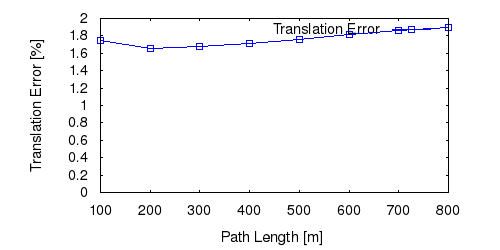This figure as: png eps pdf txt gnuplot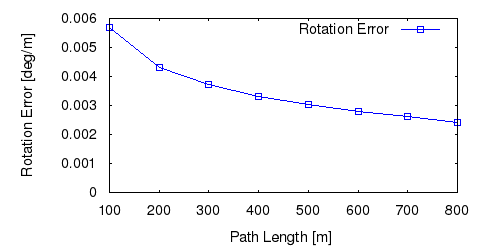This figure as: png eps pdf txt gnuplot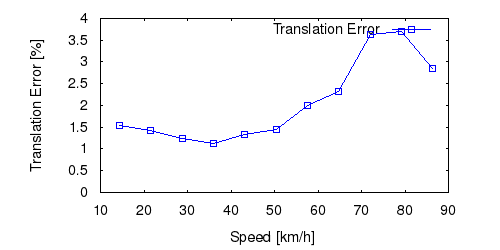This figure as: png eps pdf txt gnuplot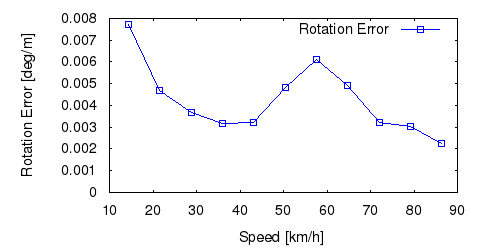This figure as: png eps pdf txt gnuplot

## Sequence 11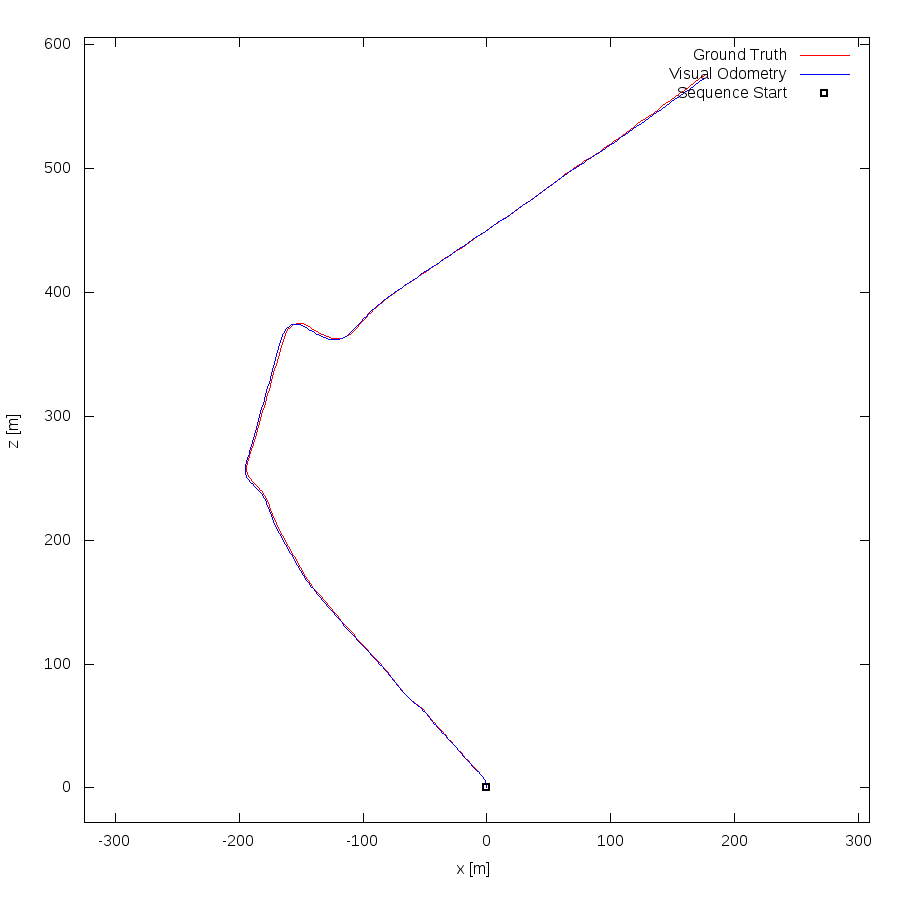This figure as: png eps pdf txt gnuplot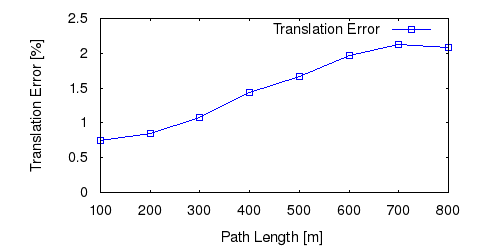This figure as: png eps pdf txt gnuplot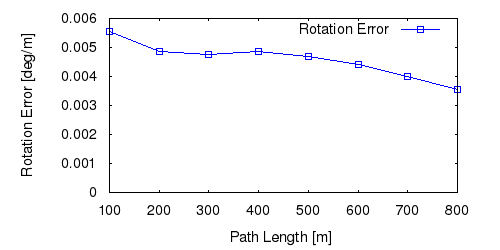This figure as: png eps pdf txt gnuplot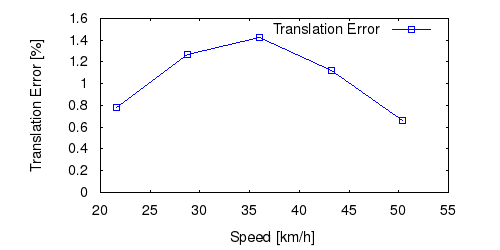This figure as: png eps pdf txt gnuplot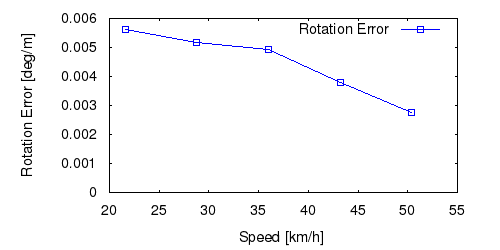This figure as: png eps pdf txt gnuplot

## Sequence 12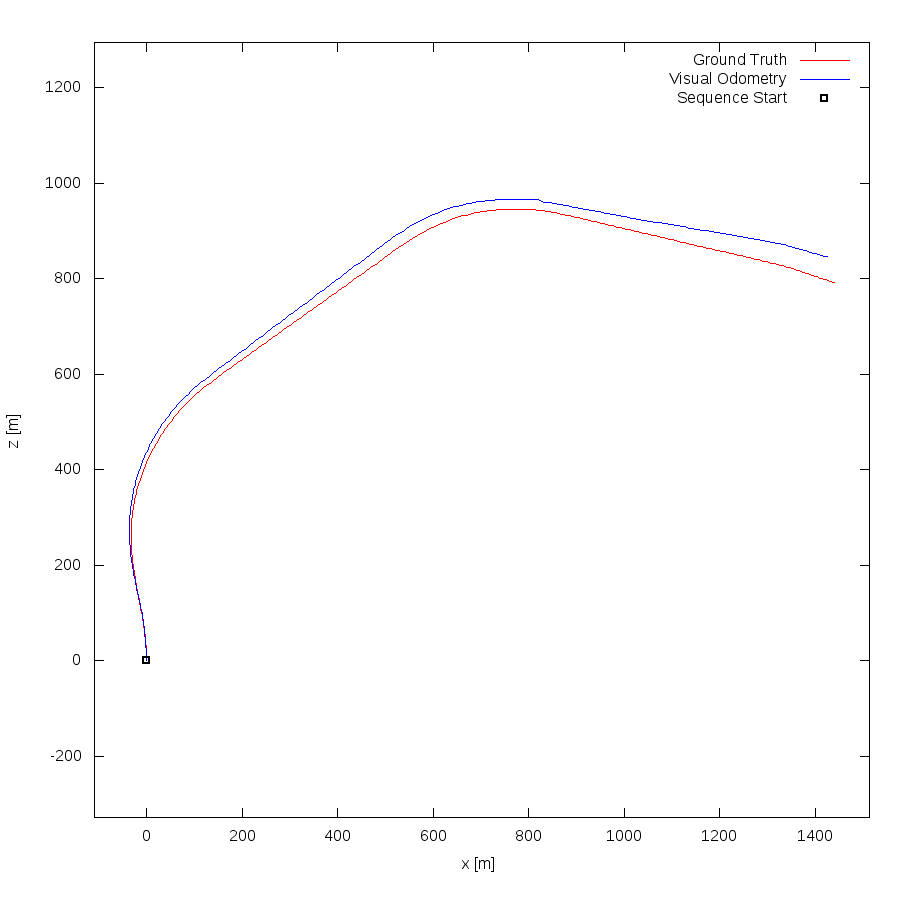This figure as: png eps pdf txt gnuplot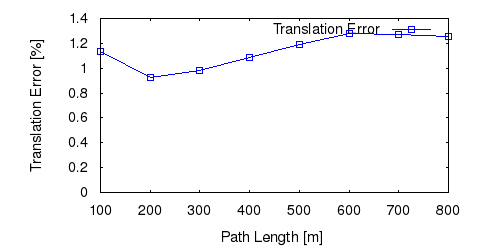This figure as: png eps pdf txt gnuplot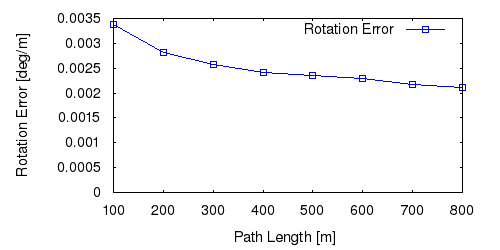This figure as: png eps pdf txt gnuplot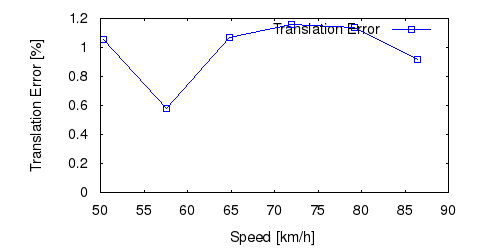This figure as: png eps pdf txt gnuplot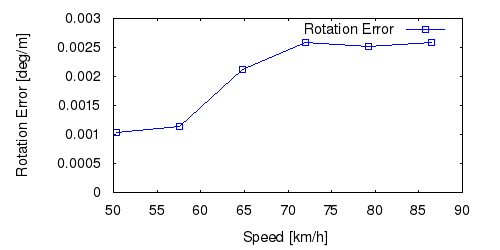This figure as: png eps pdf txt gnuplot

## Sequence 13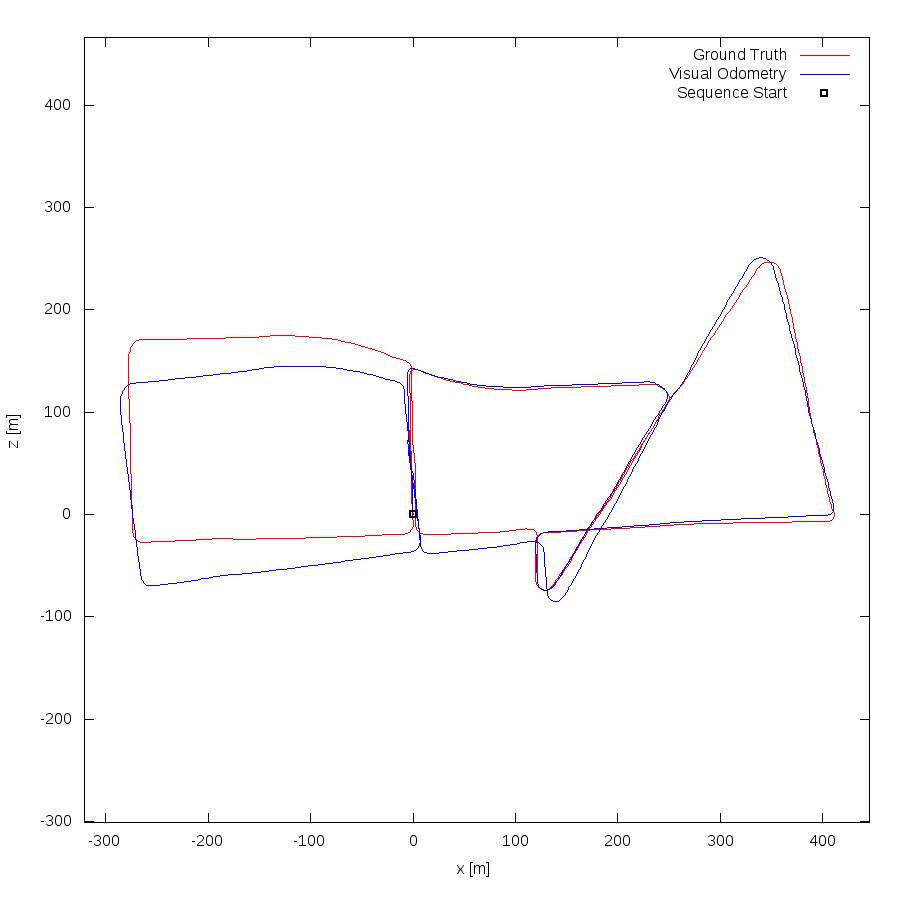This figure as: png eps pdf txt gnuplot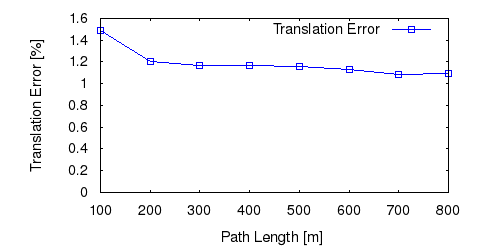This figure as: png eps pdf txt gnuplot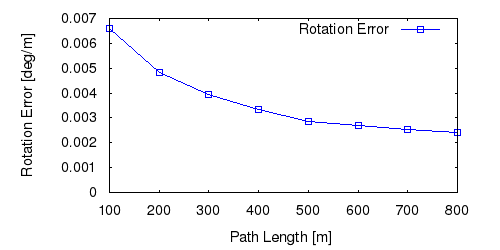This figure as: png eps pdf txt gnuplot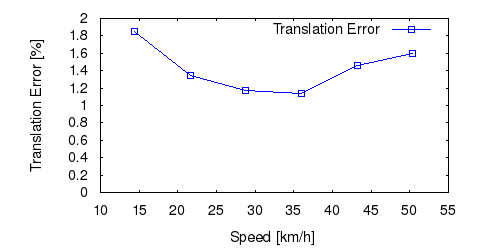This figure as: png eps pdf txt gnuplotThis figure as: png eps pdf txt gnuplot

## Sequence 14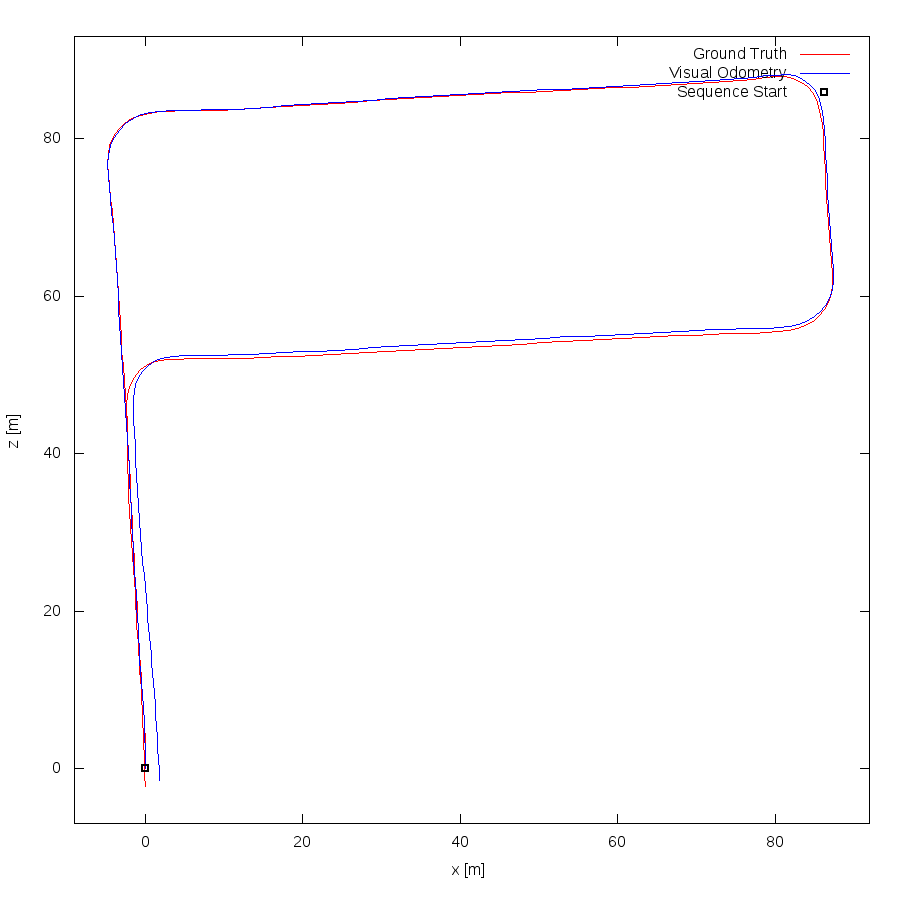This figure as: png eps pdf txt gnuplot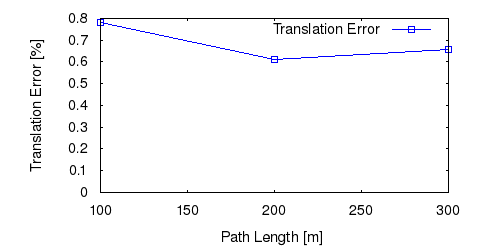This figure as: png eps pdf txt gnuplot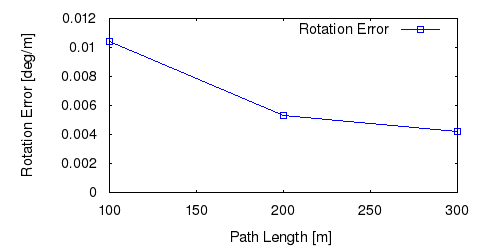This figure as: png eps pdf txt gnuplot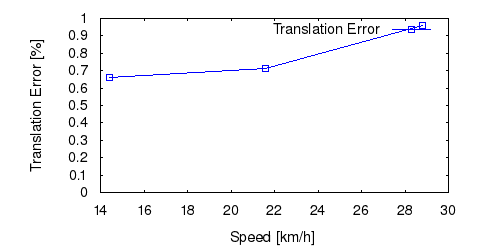This figure as: png eps pdf txt gnuplot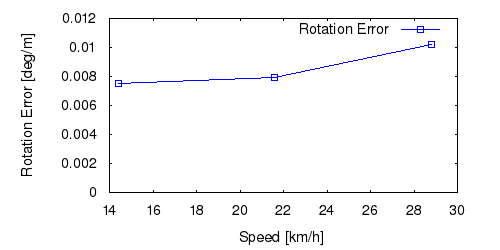This figure as: png eps pdf txt gnuplot

## Sequence 15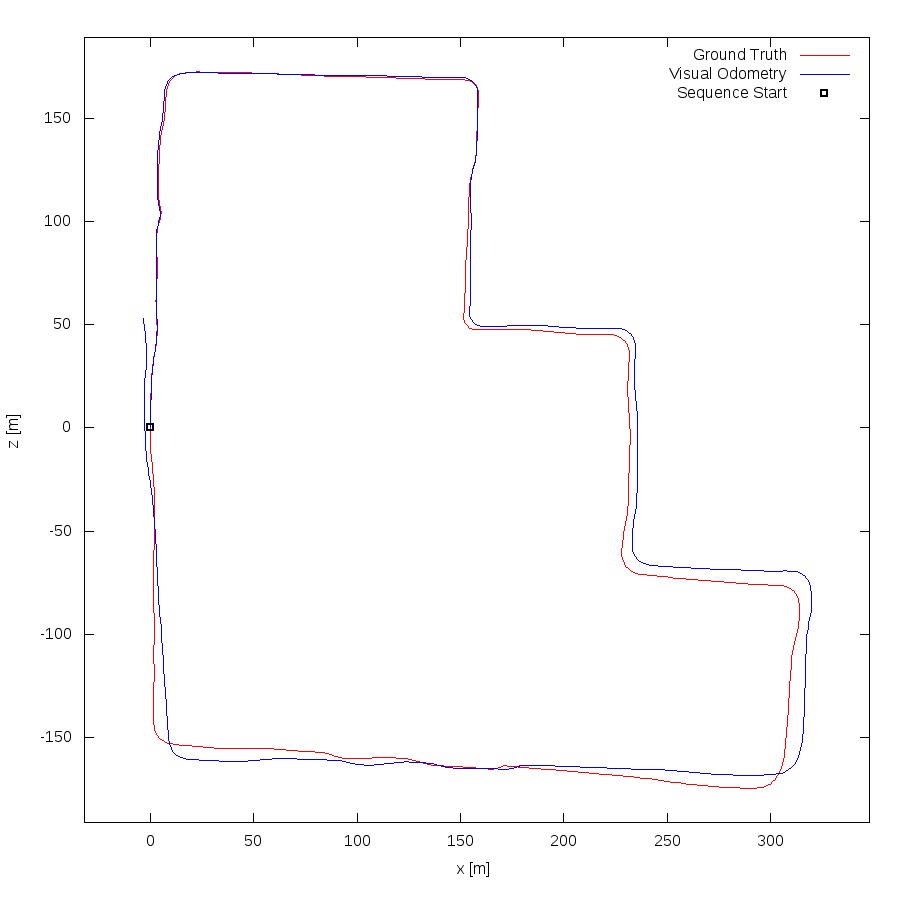This figure as: png eps pdf txt gnuplot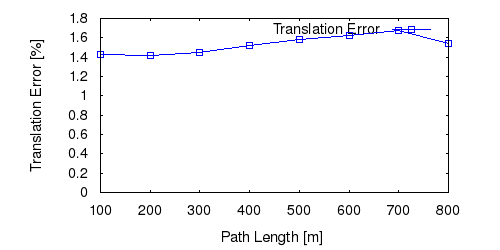This figure as: png eps pdf txt gnuplot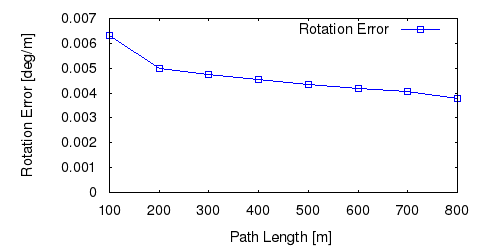This figure as: png eps pdf txt gnuplot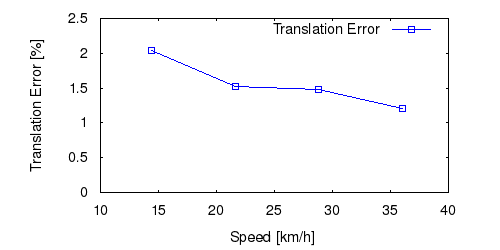This figure as: png eps pdf txt gnuplot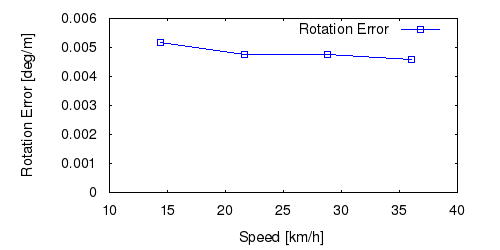This figure as: png eps pdf txt gnuplot